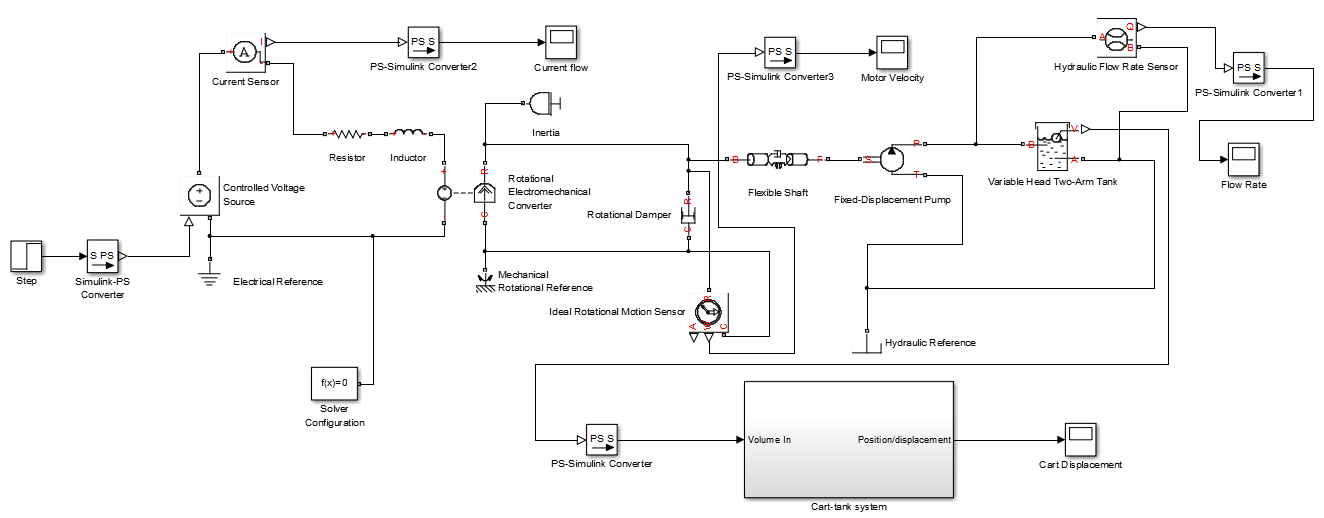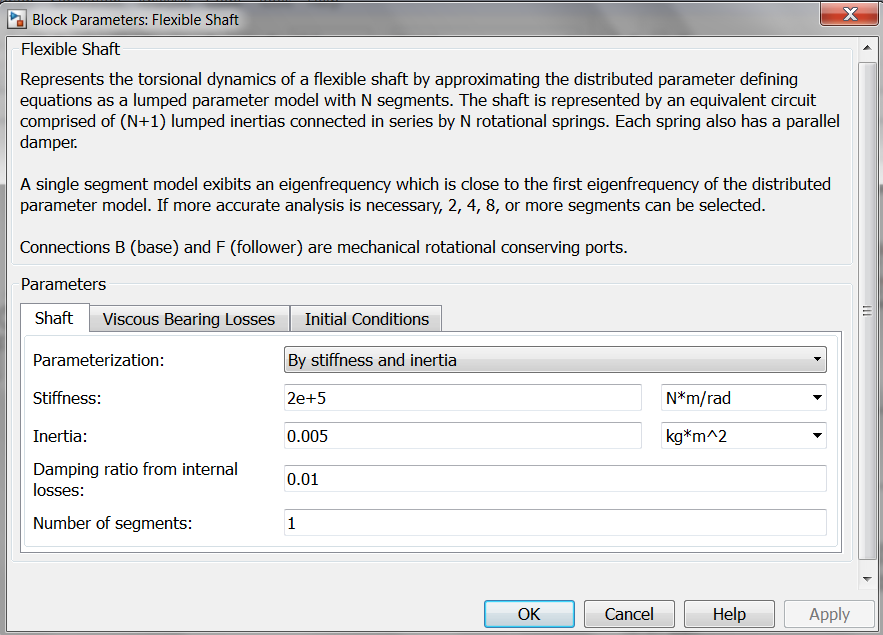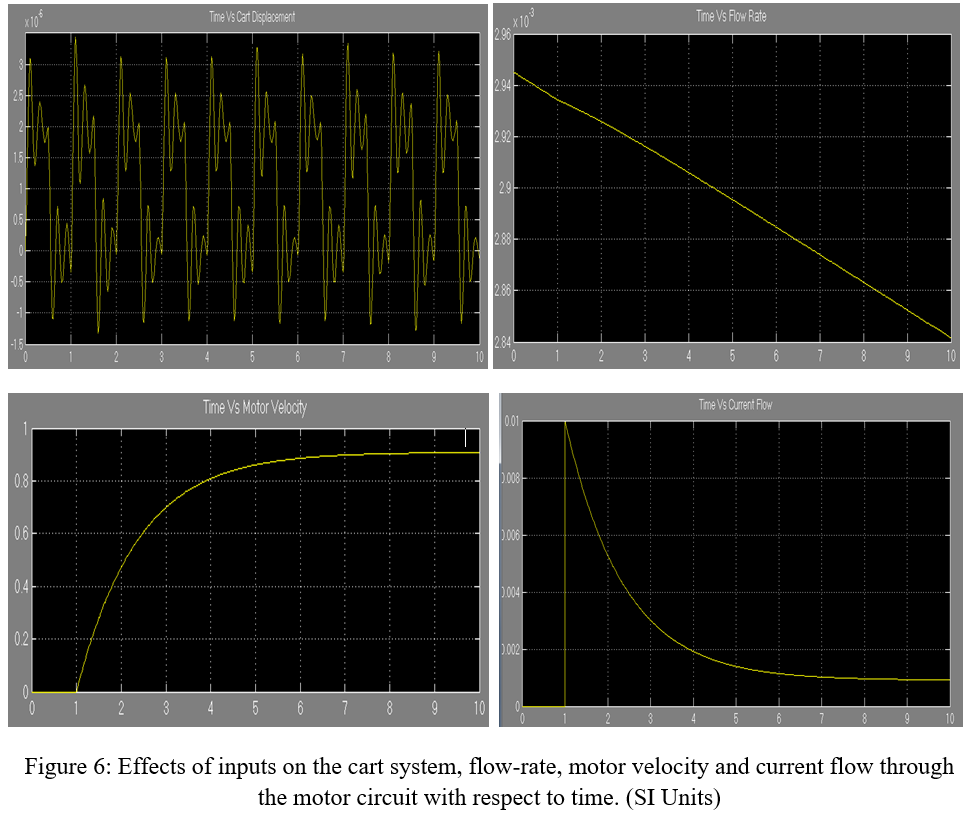## Welcome to the World of Modelling and Simulation

### What is Modelling?

This blog is all about system dynamics modelling and simulation applied in the engineering field, especially mechanical, electrical, and ...### An Electro-mechanical System Model by MATLAB SIMULINK: Part 1

The goal is to design an electro-mechanical system which is composed of the following parts:
a. a DC electric motor driven water pump
b. a water tank that is fed by the pump and that drains back into a reservoir
c. a pneumatically operated cart with spring and damper

The overall mechatronic system modelling is shown step by step in the following:Figure 1: The overall system model is represented by SIMSCAPE/SIMULINK.

## Description of the system model:

The model shown in figure 1 is built mainly by SIMSCAPE on SIMULINK environment. SIMSCAPE employs the physical network approach which basically differs from the traditional SIMULINK method. This tool is very convenient to simulate systems which consist many real physical components. The model which is developed by this tool contains mainly four basic parts. The description of each section of the model is given below:

### DC Motor:

The DC motor is an electromechanical device which converts electrical energy into mechanical energy. The motor model contains one resistor, one inductor, a rotational electromechanical converter, inertia and damping effect of the mechanical side of the motor. The parameter values for each of the block are set according to the question. Here, in addition inertia and damping of the motor are introduced. A current sensor is added to see the effect of input voltage on current in the circuit. A scope is added from SIMULINK, but a converter is added also between the current sensor and scope to convert the signal from one environment to another. Another rotational motion sensor is added to observe the angular velocity of the motor.

### Pump:

A fixed displacement pump is chosen from SIMSCAPE for the model. The pump is connected to the motor through a flexible shaft coupling which has a very low inertia and high stiffness. There are some additional parameters for the pump which are set as the default SIMSCAPE values.

### Water Tank:

A variable head two arm water tank is selected from SIMSCAPE which is connected to the pump. There are two ports. One inlet port through which water enters into the tank and one outlet port through which water drains back to the reservoir. The parameters mentioned in the question are utilized for modeling the tank. However, there are some more additional parameters for the tank block in SIMSCAPE and again the SIMSCAPE default values are set. A hydraulic sensor is also added to the tank which helps to visualize the flow rate of the water.

### The Cart-tank System:

This is the last part of the model. And, this part is done solely by SIMULINK. Figure 2 represents this model. The mass of the water is obtained first from the tank and further fed to this subsystem. This model is similar to a second order differential equation representation. The spring and damping coefficients are added by the gain blocks which are then feed back to the system. The input force is a pneumatic force which has a certain specific square shape is created by the signal builder block from SIMULINK. The output from this subsystem is the displacement/position of the cart.Figure 2: The cart-tank subsystem.

Now, through some figures following, the parameters values will be highlighted as these define the response of the dynamic system.Figure 3: Parameters for the flexible shaft coupling.Figure 4: Parameters for the pump.Figure 5: Parameters for the water tank.The change in the step input to the motor and the square wave input to the cart will have certain effects on the overall dynamic system. These will be investigated now in the following parts. The solver is selected ODE15S as this is recommended by MathWorks for simulation using SIMSCAPE.Observation 1: Step input (1 V) & Square Wave (I V, 1 HZ)Different case studies are considered to analyze the effects of the inputs on the overall system dynamics. So, first the input to the DC motor is set to 1 V (step time 1 second) and the pneumatic force on the cart-tank system is set to 1V and frequency 1 HZ. Figure 6 shows the response of the cart, flow rate in tank, motor angular velocity and current flow in the circuit with respect to time due to the step 1 V input and square wave input (1V, 1HZ) as well. From the figure, the cart displacement is extremely small and has complex periodic waveform.  The flow rate in the tank is approximately 0.003 m3/s and decreases slowly with the increase of the simulation time. The motor angular speed is transient up to approximately 5 seconds and then it gets steady state where the speed is about 0.9 rad/s. Finally the current flow is decreasing exponentially with the progress of simulation time. From the next sections, the inputs to the motor and cart as well are varied to see the effects of these responses.#ElectroMechanical #StepInput #DynamicSystem #MATLAB #SIMULINK #SIMSCAPE #Waveform #Blog #Blogger